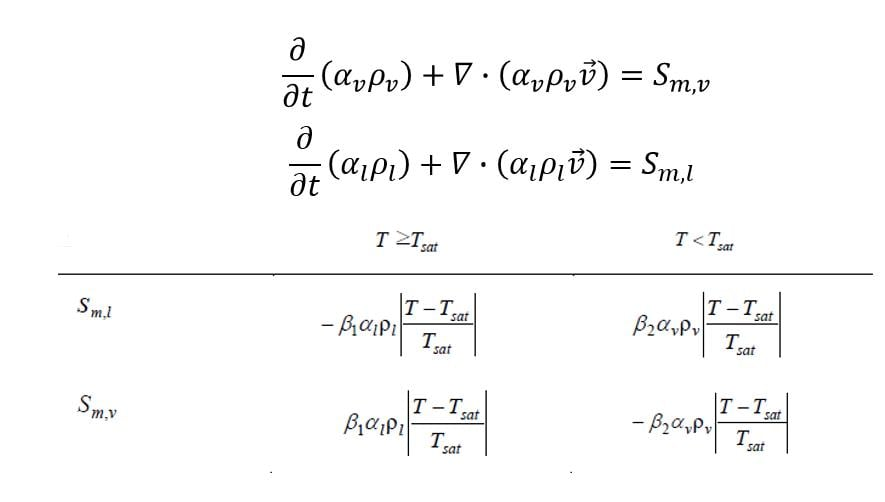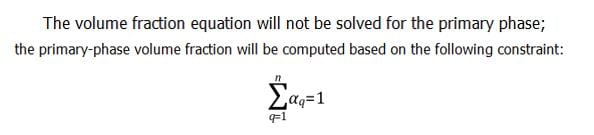## Fluids

•98546948
Subscriber

Hello everyone,

I'm doing a simulation about evaporation and condensation using VOF Model in Fluent. I compiled a UDF of primary phase for mass conservation. But It seems that the UDF doesn't work. I'm confused that whether the continuity equation of primary phase was solved at every time step?

Kindly help me out

Thank you.

•mli
Ansys Employee

•98546948
Subscriber

Thanks for your time. Actuallythe simulation was performed by Evaporation and Condensation Model of VOF Model in Fluent.  The Lee Model was used to calculated the mass transfer rate. The rate of evaporation and condensation is calculated ,respectively.  In a seal container,  the mass increase caused by evaporation is equal to that by condensation.  But it's not easy that the coefficient of evaporation and condensation is turned to match experimental data. So,  I compiled a source UDFs, which adding two equations to guarantee that as much liquid disappears, as much gas is produced.  And the source equations are described as follows:In Fluent Help, there is some describtion like this:So, I'm confused that whether the continuity equation of primary phase was solved at every time step in UDFs?

•Karthik R

Hello,

You are correct, Fluent does not solve the volume fraction equation for the primary phase. It only solves it for all the secondary phases. The volume fraction of the primary phase is obtained by subtracting the sum of secondary phase volume fractions from 1. All your source terms are appended for the secondary phase VF equations.

Thank you.

Best Regards,

Karthik

•98546948
Subscriber

Oh,It's really not a piece of good news that the source equation of primary phase be not solved.

Best wishes.

•DrAmine
Ansys Employee

The more general Eulerian Model has the option to solve N continuity equations. You might use if you think you could have better results.

•98546948
Subscriber

Thanks for your recommendation.